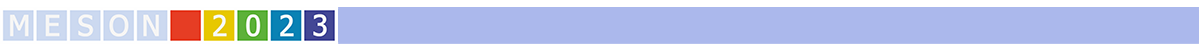#MESON2023

Jun 22 – 27, 2023
Auditorium Maximum
Europe/Warsaw timezone

## Pion absorption from the lowest atomic orbital in $^2$H, $^3$H and $^3$He

Jun 23, 2023, 5:10 PM
20m
Medium lecture hall (B) (Auditorium Maximum)

Parallel

### Speaker

Jacek Golak (Jagiellonian University)

### Description

The $\pi^- + {^2{\rm H}} \rightarrow n + n$, $\pi^- + {^3{\rm H}} \rightarrow n + n + n$, $\pi^- + {^3{\rm He}} \rightarrow n + d$ and $\pi^- + {^3{\rm He}} \rightarrow p + n + n$ capture reactions from the lowest atomic orbitals are studied under full inclusion of final state interactions. Our results are obtained with the single-nucleon and two-nucleon transition operators derived at leading order in chiral effective field theory. The initial and final three-nucleon states are calculated with the chiral nucleon-nucleon SMS potential up to N$^4$LO$^+$ augmented by the consistently regularized chiral N$^2$LO three-nucleon potential. We found that absorption rates depend strongly on the nuclear pion absorption operator used, and its two-body parts change the rates by a few orders of magnitude. The final state interactions between nucleons generated by the two-nucleon forces are also important, while the three-nucleon interaction plays a visible role only in the $\pi^- + {^3{\rm He}} \rightarrow n + d$ reaction. Our absorption rate for the $\pi^- + {^2{\rm H}} \rightarrow n + n$ process is in good agreement with the experimental data from the hadronic ground-state broadening in pionic deuterium. The capture rates on $^3{\rm He}$ are also generally consistent with the spectroscopic data within error bars, though our central values are found to be systematically below the data. We show that for the three-body breakup processes the dominant contributions to the absorption rates arise from the quasi-free scattering and final-state interaction kinematical configurations.

### Primary authors

Andreas Nogga (Forschungszentrum Jülich) Arseniy A. Filin (Ruhr-Universität Bochum) Evgeny Epelbaum (Ruhr-Universität Bochum) Henryk Witała (Jagiellonian University) Hiroyuki Kamada (Kyushu Institute of Technology) Jacek Golak (Jagiellonian University) Kacper Topolnicki (Jagiellonian University) Roman Skibiński (Jagiellonian University) Vadim Baru (Ruhr-Universität Bochum) Vitalii Urbanevych (Jagiellonian University)